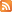Entries (RSS) -Comments (RSS)

### 9-05 Stash It Collection Tuesday February 16

Here is what I will be collecting from you on Tuesday.
1. All MathLinks including Intro and Wrap it Up.
2. One of your tests signed.
3. Journal (Hilite a place where you were having difficulty.)
4. Self Assessment
5. Foldables
6. Chapter 4 Blog Problems.

Please check out the other websites on our homepage.

### 9-06 Work to be Handed In

On Tuesday February 16th 2010 I will collect the following from you in a "Stash-it."
1. MathLink Chapter 3 Intro
2. Mathlink 3.1
3. Mathlink 3.3
4. Mathlink 3.4
5. Mathlink Wrap It Up
6. Chapter 3 Self assessment
7. Tests signed by parent.
8. Journal
All pages should be completed for 20% of this units mark.

### Chapter 3 Practice Test Answers for 9-06

BLM 3–12 Chapter 3 Test
1. D 2. C 3. D 4. A 5. D 6. 28
7. -1/8 8. –(4)^3, (–1)^5, 2^3, (–4)^2
9. a) 3 × 3 × 3 × 3 × 3 × 3 × 3
b) (–1) × (–6) × (–6) × (–6) × (–6) × (–6)
c) 4 × 4 × 4 × 5 × 5 × 5
10. a) 6^3 b) 7^4 c) 2^12
11. 11^3 means that a base of 11 is multiplied
3 times: 11^3 = 1331. 3^11 means that a base of 3
is multiplied 11 times: 3^11 = 177 147.
12. a) In the third line, Austin incorrectly distributed the exponent over subtraction to the bases of 1 and 0.05. You can only distribute an exponent over multiplication: (ab)^x = (a^x)(b^x).
I = 100(0.95)^3; I = 100(0.857375); I ≈ 86. The light intensity is approximately 86%.
b) When d = 15, I = 46%.